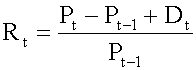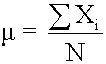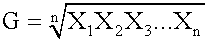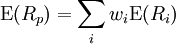Subject 1. Major Return Measures
#cfa #cfa-level-1 #has-images #portfolio-management #risk-and-return-part-i
There are various types of return measures.

Holding Period ReturnRefer to Reading 6 for a detailed discussion of this return measure.

Arithmetic or Mean ReturnRefer to Reading 7 for a detailed discussion of this return measure.

Geometric Mean ReturnRefer to Reading 7 for a detailed discussion of this return measure.

Money-Weighted Return or Internal Rate of Return

The dollar-weighted rate of return is essentially the internal rate of return (IRR) on the portfolio. Refer to Reading 6 for a detailed discussion of this return measure.

Annualized Return

Annualizing returns allows for comparison among different assets and over different time periods.

rannual = (1 + rperiod)c - 1

where c is the number of periods in a year and rperiod is the rate of return per period.

Example

Monthly return: 0.6%. The annualized return is (1 + 0.6%)12 - 1 = 7.44%.

Portfolio Return

The expected return on a portfolio of assets is the market-weighted average of the expected returns on the individual assets in the portfolio.where Rp is the return on the portfolio, Ri is the return on asset i and wi is the weighting of component asset i (that is, the share of asset i in the portfolio).

Other Major Return Measures

1. A gross return is the return before any fees, expense, taxes, etc. A net return is the return after deducting all fees and expenses from the gross return.

2. Different types of investments generate different types of income and have different tax implications. For example, in the U.S. the interest income is fully taxable at an investor's marginal tax rate while capital gains are taxed at a much lower rate. Therefore, many investors therefore use the after-tax return to evaluate mutual fund performance.

3. The nominal return and the real return are two ways to measure how well an investment is performing. The real return takes into consideration the effects of inflation when calculating how much buying power has changed.

4. An investor can also use leverage to amplify his expected return (and risk).
If you want to change selection, open original toplevel document below and click on "Move attachment"